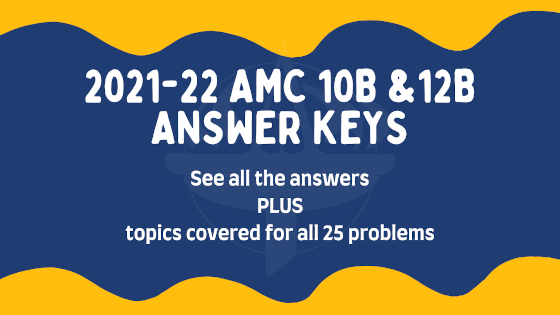# 2021-22 AMC 10B & AMC 12B Answer Key ReleasedPosted by

Yesterday, thousands of middle school and high school students participated in this year’s AMC 10B and 12B Competition. Hopefully everyone was able to take the exam safely, whether they took it online or in school!

The problems can now be discussed! See below for answer keys for both the Fall 2021-22 AMC 10B and AMC 12B questions as well as the concepts tested on each problem.

1
E
arithmetic and place values
2
B
geometry: areas
3
E
arithmetic: fractions, common denominators, and difference of two squares
4
C
algebra: equations and absolute values
5
E
arithmetic: exponents and division
6
B
number theory: prime factorizations and number of factors
7
C
algebra: fractions, completing the rectangle, and factors
8
C
number theory: primes and divisibility
9
C
algebra and arithmetic: ratios and fractions
10
A
logic and number theory: primes, perfect squares, and logical reasoning
11
B
geometry: areas, circles, regular hexagons, and equilateral triangles
12
D
algebra: equations, substitutions, and logical thinking
13
B
geometry: areas, triangles, and inscribed squares
14
C
combinatorics and number theory: probability, divisibility, and complementary counting
15
D
geometry: similar triangles and Pythagorean theorem
16
D
combinatorics: arrangements and expected values
17
D
geometry and algebra: lines and transformations
18
E
geometry: areas, regular polygons, and special angles
19
A
algebra: scientific notation, roots, and estimations
20
C
combinatorics: probability, symmetry, and conditional probability
21
E
geometry and combinatorics: polygons, angles, and patterns
22
B
number theory: modular arithmetic, sequences, and patterns
23
D
combinatorics: complementary counting, cases, and careful thinking
24
A
combinatorics: counting, symmetry, and spatial reasoning
25
E
geometry and algebra: similar triangles, lengths, and solving equations

1
E
arithmetic and place values
2
B
geometry: areas
3
C
algebra: equations and absolute values
4
E
arithmetic: exponents and division
5
C
algebra: fractions, completing the rectangle, and factors
6
C
number theory: primes and divisibility
7
D
algebra: equations, substitutions, and logical thinking
8
D
geometry: triangles, areas, and trigonometry
9
B
10
E
algebra: unit triangle trigonometry and isosceles triangles
11
C
combinatorics and number theory: probability, divisibility, and complementary counting
12
B
number theory: prime factorization and sum of divisors
13
E
algebra: trigonometry and identities
14
B
algebra: complex numbers, polynomials, and careful thinking
15
E
geometry: areas, regular polygons, and special angles
16
B
number theory: cases, GCDs, and equations
17
A
combinatorics: probability, recursion, and states
18
A
combinatorics: sequences, recursion, and limits
19
E
geometry and combinatorics: polygons, angles, and patterns
20
A
combinatorics: counting, symmetry, and spatial reasoning
21
A
algebra: complex numbers, polar form, and substitutions
22
C
geometry: triangles, circles, tangent lines, and coordinates
23
A
combinatorics: counting and expected values
24
C
geometry: triangles, circles, Power of a Point, and Stewart’s theorem
25
C
number theory: remainders, factors, and cases

In total, 11 questions of the same questions appeared on both the AMC 10B and AMC 12B. They are listed below: